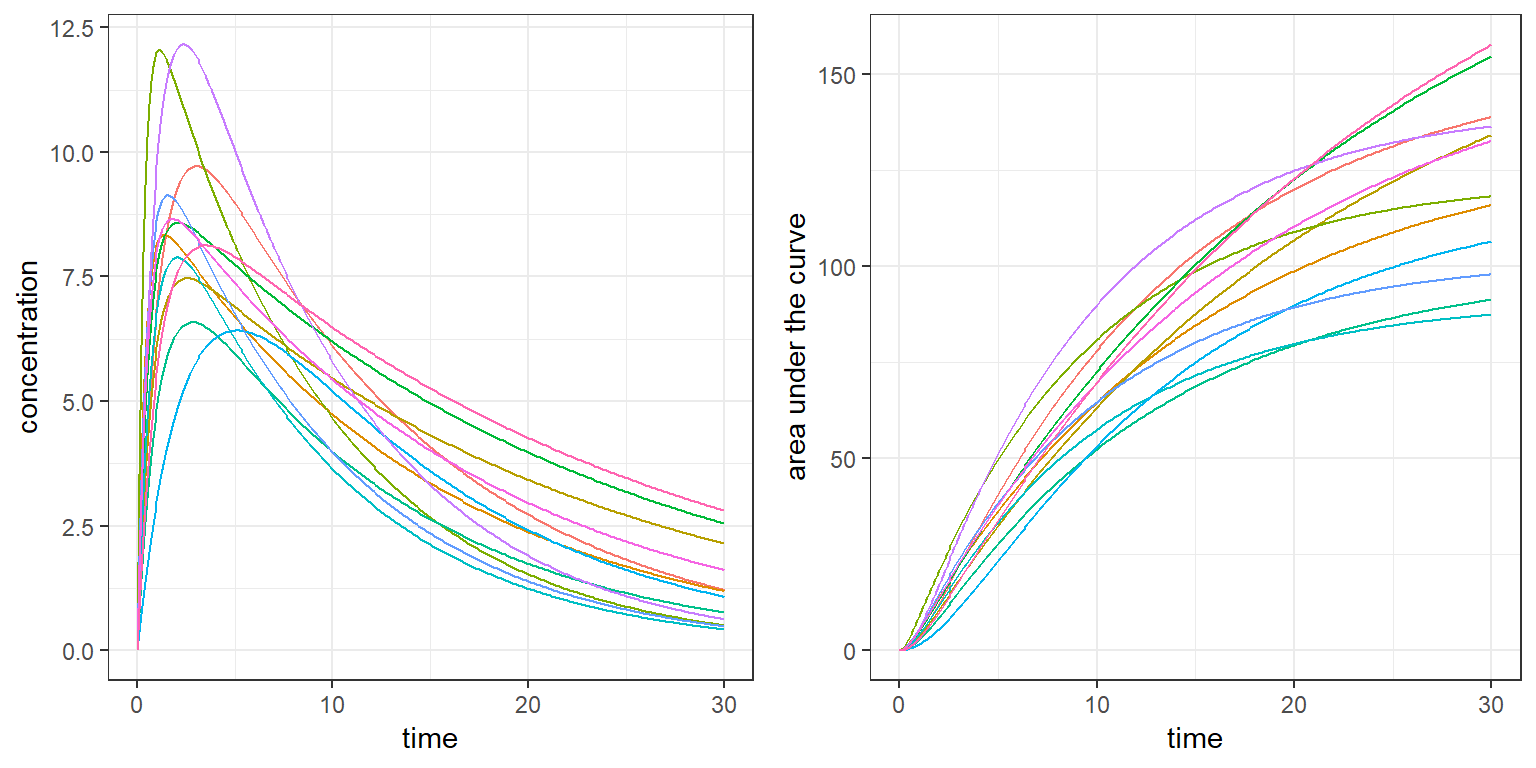R scripts: newoutput.R

Mlxtran codes: model/newoutput.txt

Monolix project: theophylline_project.mlxtran

$$\newcommand{\esp}{\mathbb{E}\left(#1\right)} \newcommand{\var}{\mbox{Var}\left(#1\right)} \newcommand{\deriv}{\dot{#1}(t)} \newcommand{\prob}{ \mathbb{P}\!(#1)} \newcommand{\eqdef}{\mathop{=}\limits^{\mathrm{def}}} \newcommand{\by}{\boldsymbol{y}} \newcommand{\bc}{\boldsymbol{c}} \newcommand{\bpsi}{\boldsymbol{\psi}} \def\pmacro{\texttt{p}} \def\like{{\cal L}} \def\llike{{\cal LL}} \def\logit{{\rm logit}} \def\probit{{\rm probit}} \def\one{{\rm 1\!I}} \def\iid{\mathop{\sim}_{\rm i.i.d.}} \def\simh0{\mathop{\sim}_{H_0}} \def\df{\texttt{df}} \def\res{e} \def\xomega{x} \newcommand{\argmin}{{\rm arg}\min_{#1}} \newcommand{\argmax}{{\rm arg}\max_{#1}} \newcommand{\Rset}{\mbox{\mathbb{R}}} \def\param{\theta} \def\setparam{\Theta} \def\xnew{x_{\rm new}} \def\fnew{f_{\rm new}} \def\ynew{y_{\rm new}} \def\nnew{n_{\rm new}} \def\enew{e_{\rm new}} \def\Xnew{X_{\rm new}} \def\hfnew{\widehat{\fnew}} \def\degree{m} \def\nbeta{d} \newcommand{\limite}{\mathop{\longrightarrow}\limits_{#1}} \def\ka{k{a}} \def\ska{k{\scriptscriptstyle a}} \def\kel{k{e}} \def\skel{k{\scriptscriptstyle e}} \def\cl{C{\small l}} \def\Tlag{T\hspace{-0.1em}{lag}} \def\sTlag{T\hspace{-0.07em}{\scriptscriptstyle lag}} \def\Tk{T\hspace{-0.1em}{k0}} \def\sTk{T\hspace{-0.07em}{\scriptscriptstyle k0}} \def\thalf{t{1/2}} \newcommand{\Dphi}{\partial_\pphi #1} \def\asigma{a} \def\pphi{\psi} \newcommand{\stheta}{{\theta^\star}} \newcommand{\htheta}{{\widehat{\theta}}}$$

Imagine that we want to define as output of simulx some variables which are not defined in the Mlxtran model code. It is then possible to create a new model file with additional lines defined in the R script.

The new input argument addlines of simulx is a list (or a list of lists) with fields * section: “[LONGITUDINAL]” (default), “[INDIVIDUAL]”, “[COVARIATE]” or “[POPULATION]” * block: “EQUATION:” or “DEFINITION:” * formula: a single string (e.g. formula = "ddt_auc=Cc"), or a vector of strings e.g. formula = c("lc = log(Cc)", "ddt_auc=Cc")

# 1 Example 1

We are using in this example the model “newoutput.txt”:

[LONGITUDINAL]
input = {V, k, a}
EQUATION:
Cc = pkmodel(V,k)
DEFINITION:
y = {distribution=lognormal, prediction=Cc, sd=a}

[INDIVIDUAL]
input = {V_pop, omega_V, w}
EQUATION:
Vpred=V_pop*(w/70)
DEFINITION:
V = {distribution=lognormal, prediction=Vpred, sd=omega_V}


Suppose that we want to compute a variable which is not defined in the model:

• the so-called “area under the curve”" $$auc(t) = \int_0^t Cc(u) du$$ where $$Cc$$ is the concentration defined in the model,

We just need for that to define the input argument addlines with an additional formula:

add1 <- list(formula=c("ddt_auc = Cc"))

adm <- list(time=seq(0,66,by=12), amount=100)
out1 <- list(name= c("Cc","auc"), time=seq(0,100, by=0.5))
p <- c(V_pop=10, omega_V=0.3, w=50, k=0.2, a=0.2)

res2 <- simulx(model     = "model/newoutput.txt",
addlines  = add1,
output    = out1,
parameter = p,
treatment = adm)

Then, auc is part of the outputs:

names(res2)
##  "Cc"        "auc"       "treatment"
head(merge(res2$Cc,res2$auc))
##   time        Cc       auc
## 1  0.0 11.031547  0.000000
## 2  0.5  9.981757  5.248911
## 3  1.0  9.031867  9.998270
## 4  1.5  8.172371 14.295832
## 5  2.0  7.394667 18.184554
## 6  2.5  6.690972 21.703141

We could also define $$Vn$$ as a new individual parameter:

add2  = list(section="[INDIVIDUAL]", block="DEFINITION:",
formula=c("Vn = {distribution=normal, prediction=Vpred, sd=1}"))

out3 <- list(name= c("Vn", "V"))
res3 <- simulx(model     = "model/newoutput.txt",
addlines  = list(add1, add2),
output    = list(out1, out3),
parameter = p,
treatment = adm,
group = list(size=5, level="individual"))
names(res3)
##  "Cc"        "auc"       "parameter" "group"     "treatment"
res3$parameter ## id Vn V ## 1 1 5.806074 10.821595 ## 2 2 7.012061 9.270943 ## 3 3 6.543728 8.575489 ## 4 4 6.204833 9.782001 ## 5 5 7.054005 5.981496 We can also create groups with different outputs which are not defined in the original model ($$\log(Cc)$$ for group 1 and $$\int Cc$$ for group2 in this example): adm1 <- list(time=seq(0,66,by=6), amount=50) adm2 <- list(time=seq(0,66,by=12), amount=100) p1 <- c(V_pop=10, omega_V=0.3, w=50, k=0.2, a=0.2) p2 <- c(V_pop=20, omega_V=0.3, w=75, k=0.1, a=0.2) y1 <- list(name="y", time=c(10,30,60)) y2 <- list(name="y", time=c(20, 50)) outc1 <- list(name = c('Cc','lc'), time=seq(0,100, by=0.5)) outc2 <- list(name = c('Cc','auc'), time=seq(0,100, by=0.5)) V <- list(name="V") add3 <- list( formula = c("lc = log(Cc)", "ddt_auc=Cc") ) g1 <- list(treatment=adm1, parameter=p1, output=list(y1,outc1), size=3, level='individual') g2 <- list(treatment=adm2, parameter=p2, output=list(y2,outc2), size=2, level='individual') res4 <- simulx(model = "model/newoutput.txt", addlines = add3, output = V, group = list(g1,g2)) names(res4) ##  "y" "Cc" "lc" "auc" "parameter" "group" ##  "treatment" # 2 Example 2 This feature is particularly useful when simulx is used with a Monolix project and when we don’t want to modify the original model used by Monolix. Computing the AUC, for instance, only requires defining this new variable in addlines: res5 <- simulx(project = 'monolixRuns/theophylline_project.mlxtran', output = list(name = c('Cc','auc'), time = seq(0, 30, by=0.1)), addlines = list(formula="ddt_auc = Cc")) names(res5) ##  "CONC" "Cc" "auc" "treatment" "originalId" ##  "population" head(merge(res5$Cc,res5$auc)) ## id time Cc auc ## 1 1 0.0 0.000000 0.0000000 ## 2 1 0.1 1.020864 0.0518490 ## 3 1 0.2 1.949228 0.2010905 ## 4 1 0.3 2.792796 0.4388658 ## 5 1 0.4 3.558635 0.7570530 ## 6 1 0.5 4.253227 1.1481971 library(gridExtra) pl1 <- ggplot() + geom_line(data=res5$Cc, aes(x=time, y=Cc, colour=id)) +
xlab("time") + ylab("concentration") + theme(legend.position="none")

pl2 <- ggplot() +  geom_line(data=res5\$auc, aes(x=time, y=auc, colour=id)) +
xlab("time") + ylab("area under the curve") + theme(legend.position="none")

grid.arrange(pl1, pl2, ncol=2)